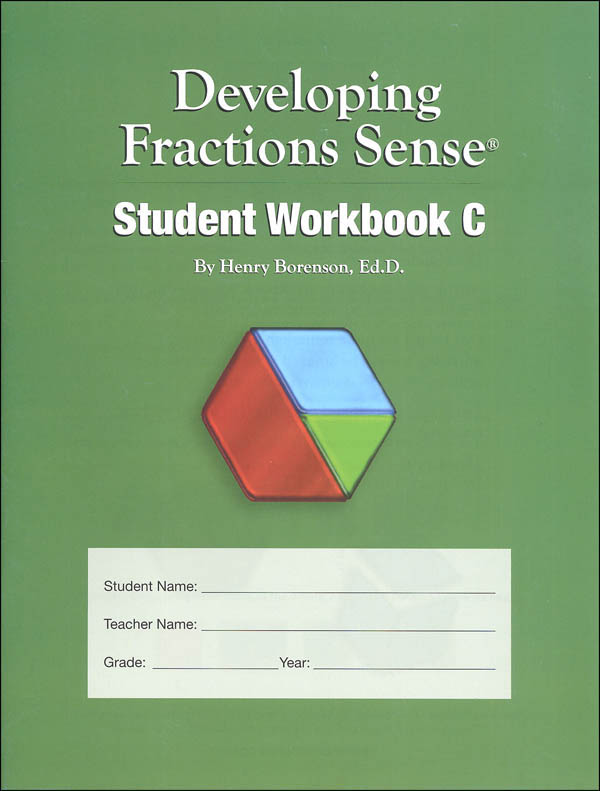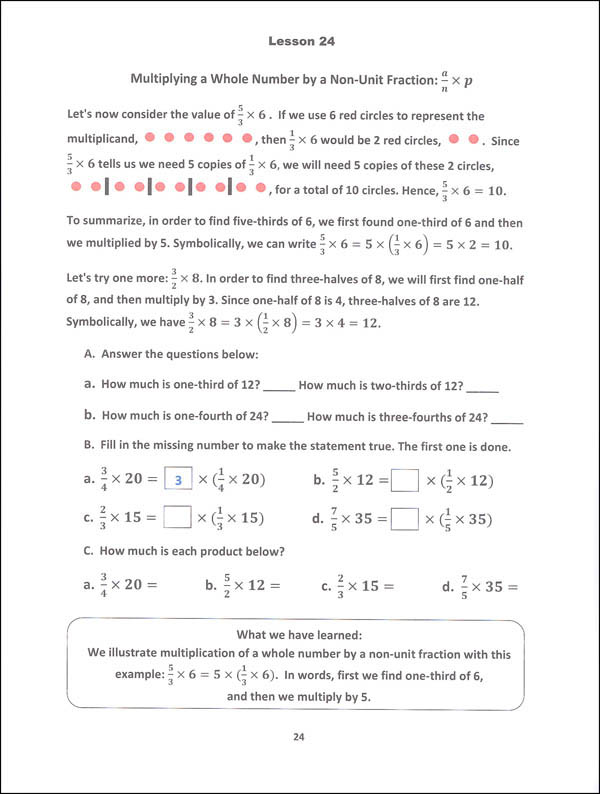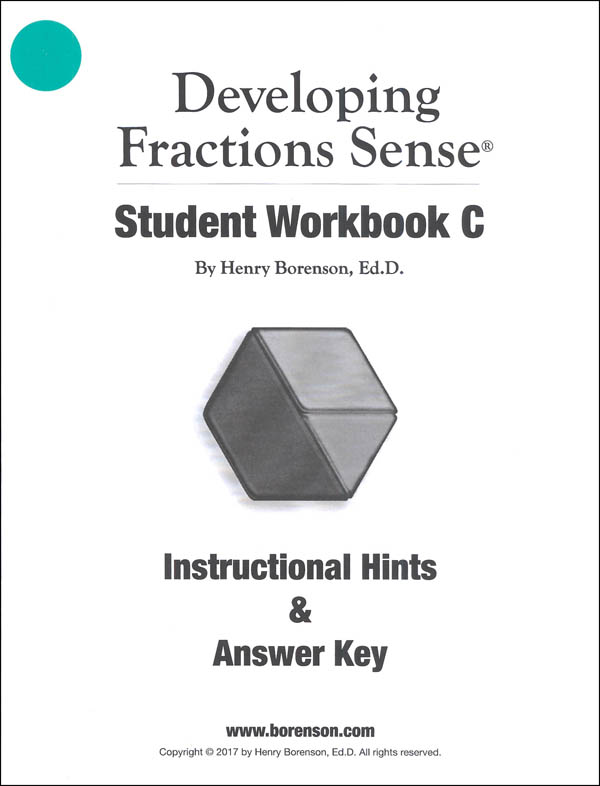••••••••# Developing Fractions Sense: Parent Set 5th Grade

# 003669

Our Price: \$13.95
2 In Stock.
Qty:
Qty:

 Item #: 003669 5

#### Product Description:

Student Workbook C is intended for 5th grade and includes 49 lessons that cover recognizing fractions and equivalent fractions, adding and subtracting fractions with different denominators and mixed fractions, solving word problems that involve fractions, understanding fractional relationships, expressing word problems as equations, multiplying and mentally multiplying a fraction by a fraction, finding the area of a rectangle with a fractional side, and understanding division of fractions.

#### Publisher Description:

Includes one copy of the Developing Fractions Sense Student Workbook C, the Instructional Hints & Answer Key booklet, and one set of plastic fraction blocks.

The Developing Fractions Sense Student Workbook C has 46 lessons, some very short, focusing on 5th grade fractions standards, and 3 enrichment lessons. All concepts are introduced via the fraction blocks before students are shown arithmetic approaches.

Category Description for Developing Fractions Sense:

Written to meet 3rd and 4th grade standards, use these sets to enhance the teaching of fractions at that level; help older students who are struggling; or use them with younger students who are ready to move ahead more quickly.

Each set includes a Student Workbook, Instructional Hints & Answer Key, and one set of plastic fraction blocks. Fractions blocks are transparent fraction tiles (3 whole hexagons, 6 halves, 9 thirds, and 15 sixths).

Student Workbook A is for 3rd grade and includes 32 lessons. Lessons are short and begin with instruction followed by exercises. Colored illustrations that match the fraction tiles accompany every explanation. Lessons are progressive and include foundational information for using this program, lines and points on number line, fractions equivalent to whole numbers; numerator and denominator; comparing fractions; adding & subtracting fractions; multiplying a fraction by a whole number and division by a unit fraction.

Student Workbook B is 4th grade and includes 45 lessons that cover recognizing/comparing/adding fractions with the same denominator; comparing & recognizing fractions; converting/adding/subtracting mixed and improper fractions; equivalent fractions; and fractions in word problems using the four functions.

Student Workbook C is intended for 5th grade and includes 49 lessons that cover recognizing fractions and equivalent fractions, adding and subtracting fractions with different denominators and mixed fractions, solving word problems that involve fractions, understanding fractional relationships, expressing word problems as equations, multiplying and mentally multiplying a fraction by a fraction, finding the area of a rectangle with a fractional side, and understanding division of fractions.

Instructional Hints & Answer Key offers suggestions for how to present the lessons; quick reference chart for the sequence of lessons;, and small format student pages with answers. Student workbooks aren't reproducible, so are available for purchase separately if working with multiple students.

Add a visual and kinesthetic component to your fraction lessons for that extra boost of understanding. ~ Donna

Primary Subject
Mathematics
5
Format
Other
Brand Name
Borenson & Associates
Weight
0.55 (lbs.)
Dimensions
11.12" x 8.38" x 0.62"